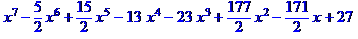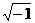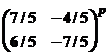WORCESTER POLYTECHNIC INSTITUTE

TWENTIETH ANNUAL INVITATIONAL MATH MEET

OCTOBER 17, 2007

TEAM EXAM QUESTION SHEET

1. A ball was floating in a lake when the lake froze. The ball was removed (without breaking the ice), leaving a hole 24 cm across the top and 8 cm deep. What was the radius of the ball in centimeters?

1. Point of tangency of two spheres described by

x2 + y2 + z2 = 121

(x-4)2 + (y-12)2 + (z-18)2  =  121

1. Consider the graphs of y = Ax2  and  y2 + 3 = x2 + 4y, where A is a positive constant and x and y are real variables.  In how many points do the two graphs intersect?

1. A certain 5 digit number has the property that if a 1 is placed after it, it is 3 times as large as with a 1 placed before it.  What is that number?

1. We have two concentric circles and wish to find the area of the annulus between them.  If we draw a chord through the outer circle tangent to the inner circle, its length is 20 inches. What is that area?

1. Factor the following polynomial over the reals as completely as possible:7.    If  z =  √2 +√2  i ,   what is z20  ?           (where  i =)

8.   Simplify  (a + b)15  mod 15.

1. Simplify  3218  mod 7.

1.  Determine the file size in Megabytes (Mb) for a digital recording made with samples of

size 2 bytes taken 44,100 times per second, in stereo, for 40 minutes. Your answer

should be rounded to the nearest tenth of a Mb.

11.   Find the sum  17 + 22 + 27+. . . +182.

12.   If it is given that

logx w=24    logy w =40     logxyz w = 12

then what is  logzw  ?     (x,y and z are all positive numbers)

13.   Simplify the following to a single fraction

-5/4 + 5/8 – 5/16 + 5/32 . . .   - 5/1024

14.   Determinewhere p is prime and positive.